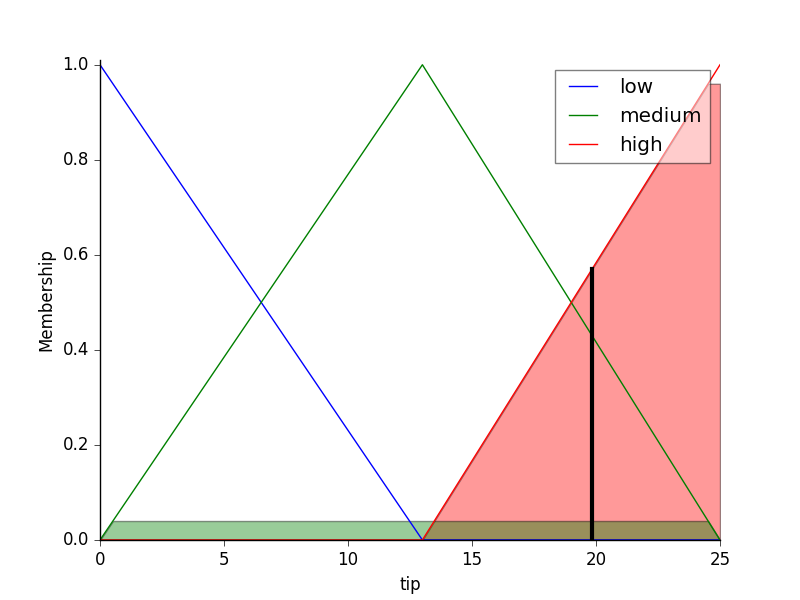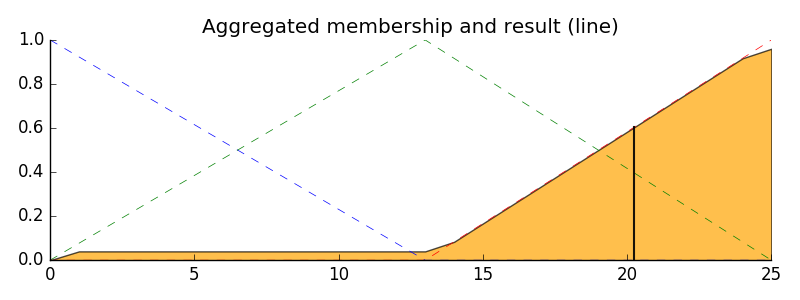# How is the fuzzy logic calculated in scikit-fuzzy?

I am following this example from documentation of scikit-fuzzy library, but cannot figure out the math behind fuzzy rules there.

Here is a short version of the example.

Food quality was 6.5 (medium)
Service was 9.8 (great)
Food quality and service can have range [0, 10]
Tip amount can have a range of [0, 25]

Rules:

1. If the food is bad OR the service is poor, then the tip will be low
2. If the service is acceptable, then the tip will be medium
3. If the food is great OR the service is amazing, then the tip will be high.

The calculated tip amount was 20.2. My question is, how is that calculated?

I was solving that by hand in this way:
Since food was "great", I applied the rule 3.
quality as % = 6.5/11 (11 is the range of rating)
service as % = 9.8/11
max(6.5/11, 9.8/11) * 26 = 23.16 (26 is the range of tip amount)

• I am not an expert in fuzzy logic, I just used it once in a paper. I think you are using an approach to calculate the tip, that may not be the same as what skfuzzy doing. Check pythonhosted.org/scikit-fuzzy/auto_examples/… May 9 at 6:20

The approach that they use proceeds something like this.

### 1. Convert quality/service membership to tip membership.

Since quality and service have no membership in the "bad"/"poor" category, there is no membership in the "low tip" category. There is .04 membership of service in the "acceptable" category, which translates to .04 membership in the "medium tip" category. Finally, quality has about .3 membership in the "great", but service has .96 in the "amazing" category. In their fuzzy logic scheme, they use Max in place of OR, so this translates to .96 membership in the "large tip" category.

### 2. Fill each tip category up to the height of their membership.

This is what is displayed in the last figure of the linked documentation.### 3. Create a single distribution by taking the OR (Max) of the membership.### 4. Find the centroid of the distribution

They mention in the link shared by Abdullah in the comments that this isn't the only way to "defuzzify" the results, but it's what they do here. I don't know how this is implemented in the code, but it can be (roughly) determined using the following formula in this case.

$$\frac{\int xP(x) \,dx}{\int P(x) \,dx}=\frac{\int_0^{13} 0.04x \,dx+\frac{1}{12}\int_{13}^{25} x(x-13) \,dx}{\int_0^{13} 0.04 \,dx+\frac{1}{12}\int_{13}^{25} (x-13) \,dx}$$

Working this out results in around 19.8 for the final defuzzified tip amount.

This gets us fairly close to the more accurate estimate from the figure in step 2, but it's not exactly the same since I have not accounted for the small excluded areas on the sides of the distribution. However, this still should give an idea of the process they are using to calculate this value.

• Wow, thank you for explaining this. However when I calculated the output this way, I got 19.8. Same when I ran python example from the documentation. 20.2 seems to be a typo then. May 10 at 7:25
• @anilewe: It is not a typo, just a difference. The document I linked divides the interval into subintervals of length 1, hence introduces some error. The newer one you linked uses smaller subintervals, and it is more accurate as a result. Also Tyberius' formula is slightly wrong. Between 0 and 13, $P(x) = \min (x/13, 0.04)$ and between 13 and 25 $P(x)=\min((x-13)/12, 0.96)$. The value of the integral does not change much, but I wanted to mention this detail. May 10 at 7:50
• Well, mine is slightly wrong too. The area is not very easy to describe, hence, $P(x)$ too. But you get the point. May 10 at 7:53
• @anilewe you are correct, the newer documentation and this integral should be closer to 19.8, which is reflected in the figure in step 2. May 10 at 12:46
• @AbdullahAliSivas you are also correct, I should have emphasized that these integrals are only roughly the described area, owing to the small excluded regions on each side. May 10 at 12:47